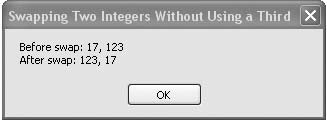# Recipe 6.4. Swapping Two Integers Without Using a Third

#### Problem

You want to swap the values of two integer variables without creating a third.

#### Solution

Sample code folder: Chapter 06\IntegerSwap

Use the exclusive-or bit manipulation function to do the trick.

#### Discussion

Nowadays efforts to save the space of a single variable in memory seem kind of silly, but this recipe nevertheless demonstrates an interesting technique for swapping two numbers without creating a third variable. More importantly, it demonstrates how bit-manipulation functions can be quite useful in Visual Basic 2005. Here's the sample code:

` Dim result As String Dim firstValue As Integer Dim secondValue As Integer ' ----- Set the initial test values. firstValue = 17 secondValue = 123 result = String.Format("Before swap: {0}, {1}", _    firstValue, secondValue) result &= vbNewLine ' ----- Swap the values at the bit level. firstValue = firstValue Xor secondValue secondValue = firstValue Xor secondValue firstValue = firstValue Xor secondValue result &= String.Format("After swap: {0}, {1}", _    firstValue, secondValue) MsgBox(result) `

The above code loads values into integers firstValue and secondValue, then swaps their values by applying three successive Xor operators on them. The Xor operator combines the two integers on a bit-by-bit basis, resulting in a 1 bit whenever the original bits are different and a 0 when they are the same. Once these three Xor operations have been performed, the original contents of the two integers will have migrated to the opposite locations in memory. Figure 6-4 shows the results displayed by the sample code.

##### Figure 6-4. Swapping two integers using XorVisual Basic 2005 Cookbook: Solutions for VB 2005 Programmers (Cookbooks (OReilly))
ISBN: 0596101775
EAN: 2147483647
Year: 2006
Pages: 400

Similar book on Amazon